# Python datetime | Date and Time in Python

This article is created to provide you with all the things that relate to the handling of dates and times in Python programming. In this article, I've included a lot of programs and their output to provide you with a better understanding of the topic in an efficient way.

## The datetime module

The "datetime" module in Python is used to work with dates and times.

## Print the current date and time in Python

The program given below finds and prints the current date and time using the "now()" method of the "datetime" attribute of the "datetime" module.

```import datetime

current_date_time = datetime.datetime.now()
print("The Current Date/Time =", current_date_time)```

The image below displays an example of the output this Python program produced: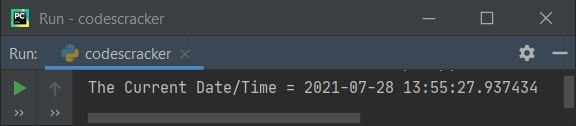In the above sample output:

1. 2021 is the year.
2. 07 is the month number, which equals "July."
3. Because 28 is the month's date, it equals the 28th of July.
4. 13 is the hour, which corresponds to 1 PM.
5. 55 is the minute time, which equals 1.55 PM.
6. 27 is the second time, which equals 1.55.27 PM.
7. 937434 is the microsecond time, which equals 1.55.27.937434 PM.

## Get all attributes of the "datetime" module

To get all the attributes available in the "datetime" module, use the "dir" method to fetch and print like shown in the program given below:

```import datetime

print(dir(datetime))```

The snapshot given below is nothing but the sample output produced by the above program: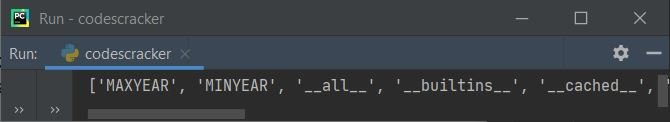Because all of the datetime module's attributes cannot be shown in a single snapshot, I've listed them all below:

• MAXYEAR
• MINYEAR
• __all__
• __builtins__
• __cached__
• __doc__
• __file__
• __name__
• __package__
• __spec__
• date
• datetime
• datetime_CAPI
• sys
• time
• timedelta
• timezone
• tzinfo

After viewing its sample output, the program below uses all of the above attributes to give you an idea of what they are used for. Let's have a look at the program first, as given below:

```import datetime

print("1.", datetime.MAXYEAR)
print("2.", datetime.MINYEAR)
print("3.", datetime.__all__)
print("4.", datetime.__builtins__)
print("5.", datetime.__cached__)
print("6.", datetime.__doc__)
print("7.", datetime.__file__)
print("9.", datetime.__name__)
print("10.", datetime.__package__)
print("11.", datetime.__spec__)
print("12.", datetime.date)
print("13.", datetime.datetime)
print("14.", datetime.datetime_CAPI)
print("15.", datetime.sys)
print("16.", datetime.time)
print("17.", datetime.timedelta)
print("18.", datetime.timezone)
print("19.", datetime.tzinfo)```

And here is its sample output: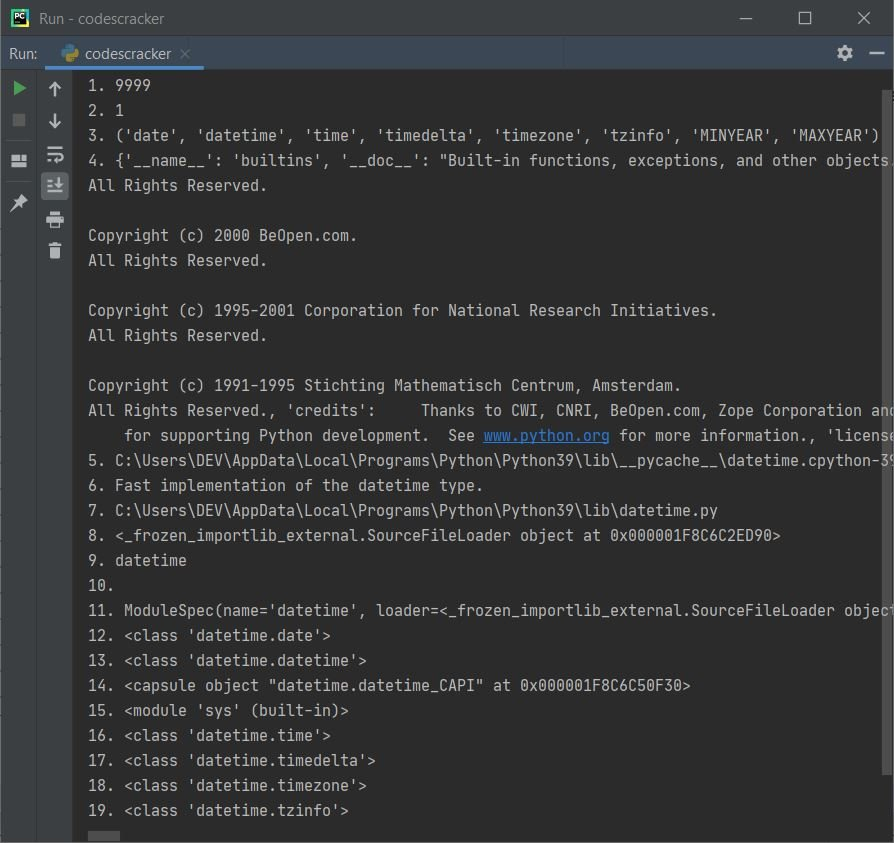## Count the total number of seconds since 1970

The following program counts the total number of ticks (seconds) that have occurred between 12:01 a.m. on July 28, 1970 and now (1:22:49 p.m. on July 28, 2021):

```import time

ticks = time.time()
print("Number of Ticks since 12:00AM, January 1970 is", ticks)```

The output produced by this program is shown in the snapshot given below: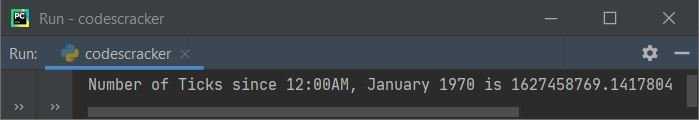### Count the number of seconds that have passed since today's midnight

This program counts total number of seconds elapsed since today's midnight, that is 12.00 AM.

```import datetime

midnight = datetime.datetime.now().replace(hour=0, minute=0, second=0, microsecond=0)
current_time = datetime.datetime.now()
seconds_elapsed = current_time - midnight
print("Total Seconds elapsed since Midnight =", seconds_elapsed.seconds)```

Here is an illustration of the output this program produced: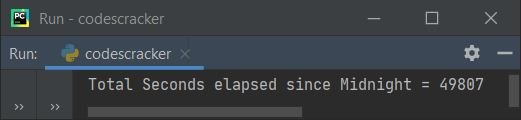Note: Since the current time is around 1.50.02, you saw this output. If you convert these seconds into hours, then you will get approx. 13 hours plus 50 minutes, then some seconds and microseconds.

### Count the total seconds required to execute any code in Python

The program given below counts the total time taken to execute the code written between the "code start" and "code end" comment lines. That is the code, print("Hello World").

```import datetime

start_time = datetime.datetime.now()

# code start
print("Hello World")
# code end

end_time = datetime.datetime.now()
total_time = end_time - start_time
print("Total time taken =", total_time)```

The snapshot given below shows the sample output:We've done the job of counting the total amount of time taken to execute the code by:

• I've initialized the current time in the variable named start_time.
• Execute the code.
• Again, I've initialized the current time, but to a different variable named end_time.
• Then I've subtracted the value of start_time from end_time and initialized the subtraction result to a new variable named total_time.
• Now prints the variable's value, which is total_time's value.
• The output will be whatever time it takes to execute the code between these two codes in the above program, start_time = datetime.datetime.now() and end_time = datetime.datetime.now().

Since the program given above is very simple and only prints a short string, the time taken is 0. Now, let's create another program that takes some 1, 2, or even more seconds to execute the code in between.

```import datetime

start_time = datetime.datetime.now()

print("Enter the limit: ")
lim = int(input())
for i in range(lim):
print(i)

end_time = datetime.datetime.now()
time_taken = end_time - start_time
print("Total time taken =", time_taken)```

Here is the initial output produced by above program: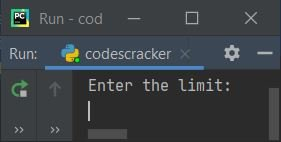Now provide the input, say 20, to execute the loop and print the value from 0 to 19. While printing all these values, the number of time taken to evaluate the loop will get printed on the output after printing these values, as shown in the snapshot given below: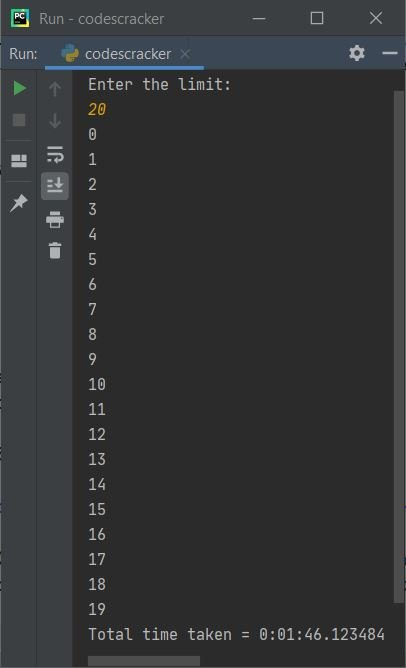### Simple Date Arithmetic

This program shows the simple arithmetic handling of dates in Python.

```import datetime

today = datetime.date.today()
print("Today's Date is", today)

yesterday = today - datetime.timedelta(days=1)
print("Yesterday's Date was", yesterday)

tomorrow = today + datetime.timedelta(days=1)
print("Tomorrow's Date will be", tomorrow)

print("Time difference between Yesterday and Tomorrow is", tomorrow - yesterday)```

The snapshot given below shows the sample output of this program:## Find the time difference

The program given below computes the time difference between current time and past time.

```from datetime import datetime

now = datetime.now()
then = datetime(2021, 7, 23)

time_difference = now - then
print("Time Difference =", time_difference)
print("Time Difference (in days) =", time_difference.days)
print("Time Difference (in seconds) =", time_difference.seconds)```

Here is its sample output. The current date is 28/07/2021, and the current time is 16:42:32.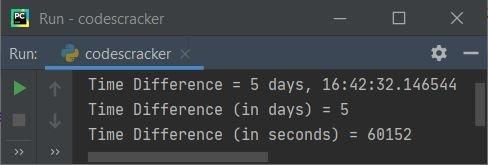#### Calculate the time difference between now and the given time

This is a modified version of the previous program. This program allows the user to define the date at run-time to make the program work in a dynamic way. That is, whatever date is provided by the user, the program finds and prints the time difference between the current date and the given date, as shown in the program and its sample run given below:

```from datetime import datetime

print("Enter Date (in DD/MM/YYYY) format: ")
mydate = input()

myday = mydate[0:2]
mymonth = mydate[3:5]
myyear = mydate[6:10]

myday = int(myday)
mymonth = int(mymonth)
myyear = int(myyear)

now = datetime.now()
then = datetime(myyear, mymonth, myday)

time_difference = now - then

print("Time Difference =", time_difference)
print("Time Difference (in days) =", time_difference.days)
print("Time Difference (in seconds) =", time_difference.seconds)```

Here is its initial sample output: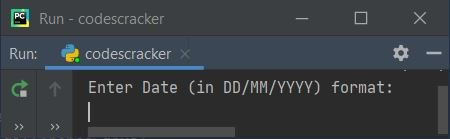Now provide the date, I've provided the date as "10/07/2021" and pressed the ENTER key. Here is the output produced: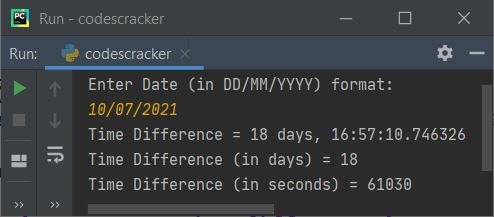Note: Whatever the user enters, the input() method of Python treats it as a string. Therefore, using string slicing, I've sliced the values of day, month, and year. That is, 10/07/2021 inputs get initialized to the "mydate" variable in a way that:

• mydate = '1'
• mydate = '0'
• mydate = '/'
• mydate = '0'
• mydate = '7'
• mydate = '/'
• mydate = '2'
• mydate = '0'
• mydate = '2'
• mydate = '1'

Now the code mydate[0:2] means the character from the 0th (included) index to the 2nd (excluded) index gets sliced. After slicing the value, we converted it to integer form with the int() method before proceeding with the job.

### Find the Next Date Following the Given Days

This program receives a number of days as input from the user at run-time to find and print the date after a given number of days. For example, if the user enters 46 days as input, then the program will calculate and print the date that will come after 46 days from now. Let's have a look at the program given below:

```from datetime import datetime, timedelta

print("Enter Total Number of Days: ")
myday = int(input())

date_after_myday = datetime.now() + timedelta(days = myday)
print("\nDate after", myday, "Days =", date_after_myday)```

Since the current date is July 28, 2021, the program produces the following output after receiving 46 days as input: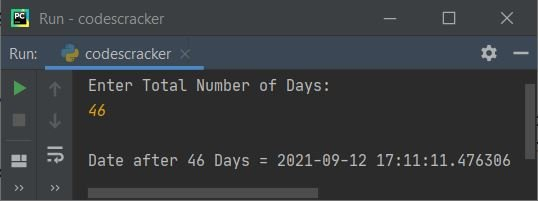Note: You are free to format the date using the date format code. The code to format the date is given at the end of this article.

### Find a Previous Date with the Days Given

Now this is the reverse of the previous program. To find the previous date with the given number of days, just replace the following line of code:

`date_after_myday = datetime.now() + timedelta(days = myday)`

from the previous program, with the code given below:

`date_after_myday = datetime.now() - timedelta(days = myday)`

With a simple + (plus) and - (minus), the entire program can be reversed. Here is the modified and complete version of the program:

```from datetime import datetime, timedelta

print("Enter Total Number of Days: ", end="")
myday = int(input())

prev_date = datetime.now() - timedelta(days = myday)
print("\nDate before", myday, "Days = ", end="")
print(prev_date.strftime("%d"), end=" ")
print(prev_date.strftime("%b"), end=", ")
print(prev_date.strftime("%Y"))```

Here is its sample output after receiving 12 as input. The current date is July 28, 2021.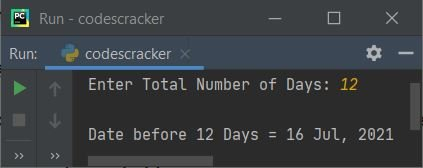### Iterate Over a Range of Dates

The program given below iterates over a range of dates. In this program, I've specified 1 as the day to iterate over, which means that the date is incremented by 1 day from the current date to the next 10 days.

```import datetime

dd = datetime.timedelta(days=1)
start_date = datetime.date.today()
end_date = start_date + (10*dd)
for i in range((end_date - start_date).days):
print(start_date + i*dd)```

Here is its sample output: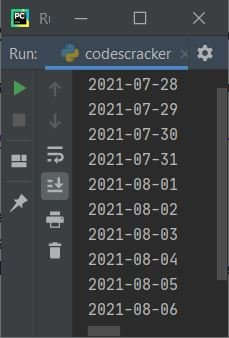## Date and Time Format Codes with Description

This section contains descriptions for all of the most important and useful date and time formatting codes. The example program for all these codes is also given after the table.

Format Code Used for
%a weekday abbreviation such as Mon, Tue
%A full version of weekdays such as Monday, Tuesday
%d Month day (01-31), for example, 01, 02
%b Month abbreviations such as Jan, Feb
%B Full month names, such as January and February
%m Month as a number (01-12), such as 01, 02
%y Year abbreviations such as 20, 21
%Y Full versions of years such as 2020 and 2021
%H For hours 00-23, such as 00 (12 AM), 01 (1 AM), 13 (1 PM), and 20 (8 PM)
%I For hours 00-12, such as 01, 02
%p For AM/PM
%M For minute (00-59), such as 00, 01
%S For second (00-59), such as 00, 01
%f For microseconds (000000-999999), such as 000000 and 000001
%j The year's day number (001-366), for example, 001, 002
%c Local version of the date and time, such as "Wed Jul 28 01:58:00 2021"
%x Local version of the date (MM/DD/YY), such as 07/28/2021, 08/26/2021
%X Local version of the time (HH:MM:SS), such as 13:59:00

The program given below uses all the above-listed directives with their examples in one single program:

```import datetime

cc = datetime.datetime.now()

print("Short Weekday =", cc.strftime("%a"))
print("Full Weekday =", cc.strftime("%A"))
print("Day of Month =", cc.strftime("%d"))
print("Short Month Name =", cc.strftime("%b"))
print("Full Month Name =", cc.strftime("%B"))
print("Month Number =", cc.strftime("%m"))
print("Short Year =", cc.strftime("%y"))
print("Full Year =", cc.strftime("%Y"))
print("Hour (in 24-hour Format) =", cc.strftime("%H"))
print("Hour (in 12-hour Format) =", cc.strftime("%I"))
print("AM/PM =", cc.strftime("%p"))
print("Minute =", cc.strftime("%M"))
print("Second =", cc.strftime("%S"))
print("Microsecond =", cc.strftime("%f"))
print("Day Number of Year =", cc.strftime("%j"))
print("Local Date/Time =", cc.strftime("%c"))
print("Local Date =", cc.strftime("%x"))
print("Local Time =", cc.strftime("%X"))```

The image below shows an example of the output this Python program produces: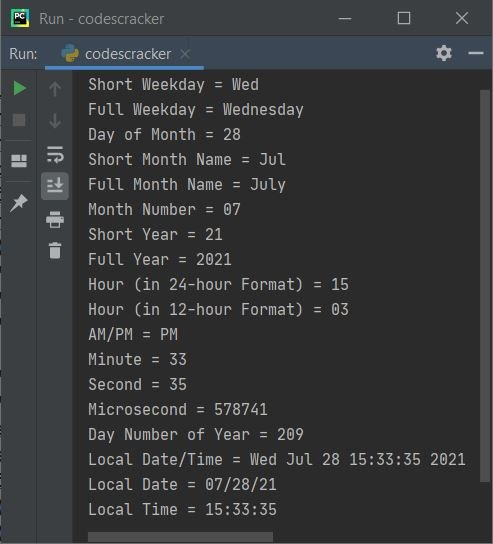Note: The strftime() method of a datetime object is used to format date objects into understandable and commonly used date and time formats. This method takes one parameter that is used to format the string (date/time) like shown in the sample program and its sample output given above.

Python Online Test

« Previous Tutorial Next Tutorial »